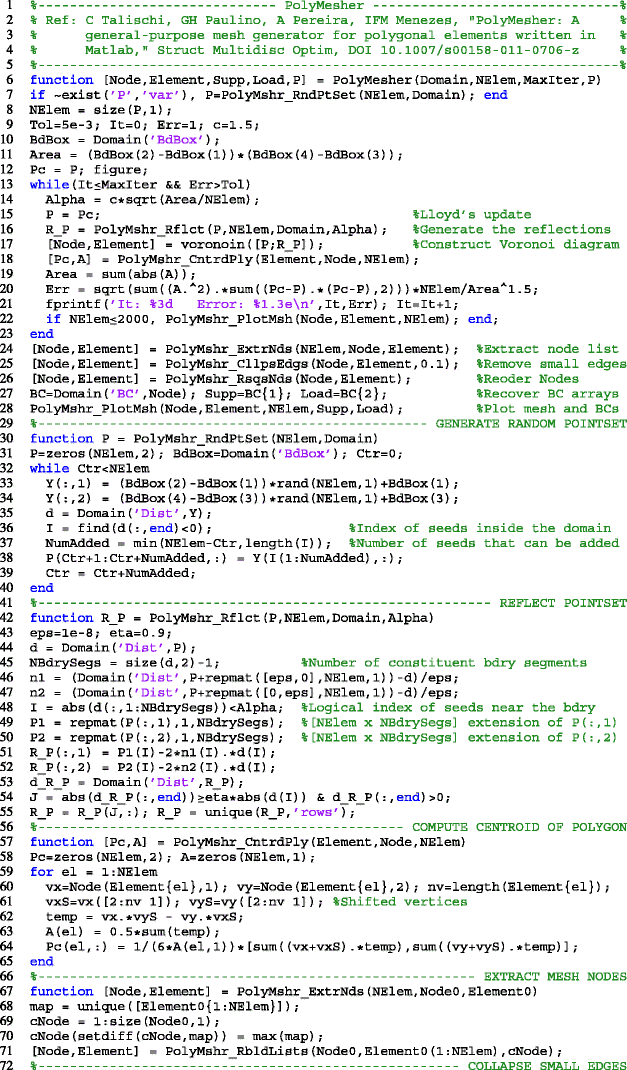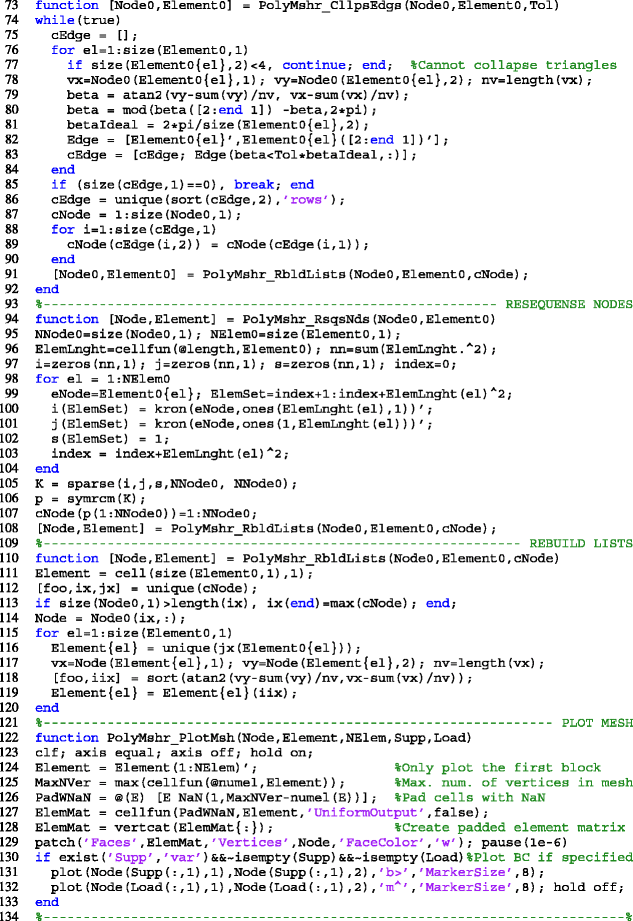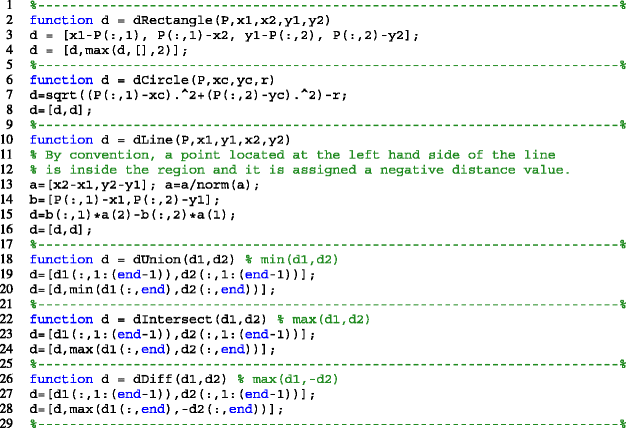# PolyMesher: a general-purpose mesh generator for polygonal elements written in Matlab

## Abstract

We present a simple and robust Matlab code for polygonal mesh generation that relies on an implicit description of the domain geometry. The mesh generator can provide, among other things, the input needed for finite element and optimization codes that use linear convex polygons. In topology optimization, polygonal discretizations have been shown not to be susceptible to numerical instabilities such as checkerboard patterns in contrast to lower order triangular and quadrilaterial meshes. Also, the use of polygonal elements makes possible meshing of complicated geometries with a self-contained Matlab code. The main ingredients of the present mesh generator are the implicit description of the domain and the centroidal Voronoi diagrams used for its discretization. The signed distance function provides all the essential information about the domain geometry and offers great flexibility to construct a large class of domains via algebraic expressions. Examples are provided to illustrate the capabilities of the code, which is compact and has fewer than 135 lines.

This is a preview of subscription content, access via your institution.

1. 1.

For example, consider $$\Omega_{1}=\left\{ \left(x_{1},x_{2}\right)\in\mathbb{R}^{2}:x_{1}<0\right\}$$ and $$\Omega_{2}=\left\{ \left(x_{1},x_{2}\right)\in\mathbb{R}^{2}:x_{2}<0\right\}$$. The formula $$d_{\Omega_{1}\cup\Omega_{2}}(\mathbf{x})=\min\left(d_{\Omega_{1}}(\mathbf{x}),d_{\Omega_{2}}(\mathbf{x})\right)$$ has incorrect distance “value” in the third quadrant, i.e., for x 1 < 0, x 2 < 0. In this region, the closest boundary point is the new corner x = (0, 0) formed by the union operation.

2. 2.

A tessellation or tiling of Δ is a collection of open sets S i such that $$\cup_{i}\overline{S}_{i}=\overline{\Delta}$$ and S i  ∩ S j  = ∅ if i ≠ j.

3. 3.

The cell structure allows for storing vectors of different size and is therefore suitable for connectivity of polygonal elements with different number of nodes.

4. 4.

This small overhead can be removed after a few iterations once a good estimate value is obtained.

5. 5.

Since the gradation in mesh is often dictated by the geometry of the domain, it is natural that both μ and h be defined based on the distance function d Ω.

## References

1. Allaire G, Jouve F (2005) A level-set method for vibration and multiple loads structural optimization. Comput Methods Appl Mech Eng 194(30–33):3269–3290. doi:10.1016/j.cma.2004.12.018

2. Allaire G, Pantz O (2006) Structural optimization with FreeFem+ +. Struct Multidisc Optim 32(3):173–181. doi:10.1007/s00158-006-0017-y

3. Andreassen E, Clausen A, Schevenels M, Lazarov B, Sigmund O (2011) Efficient topology optimization in MATLAB using 88 lines of code. Struct Multidisc Optim 43(1):1–16. doi:10.1007/s00158-010-0594-7

4. Aurenhammer F (1991) Voronoi diagrams—a survey of a fundamental geometric data structure. ACM Comput Surv 23(3):345–405

5. Bolander JE, Saito S (1998) Fracture analyses using spring networks with random geometry. Eng Fract Mech 61(5–6):569–591. doi:10.1016/S0013-7944(98)00069-1

6. Challis VJ (2010) A discrete level-set topology optimization code written in matlab. Struct Multidisc Optim 41(3):453–464. doi:10.1007/s00158-009-0430-0

7. Cuthill E, McKee J (1969) Reducing the bandwidth of sparse symmetric matrices. In: Proceedings of the 24th national conference. ACM Press, New York, NY, pp 157–172. doi:10.1145/800195.805928

8. Du Q, Gunzburger M (2002) Grid generation and optimization based on centroidal Voronoi tessellations. Appl Math Comput 133(2–3):591–607. doi:10.1016/S0096-3003(01)00260-0

9. Du Q, Wang DS (2005) The optimal centroidal Voronoi tessellations and the Gersho’s conjecture in the three-dimensional space. Comput Math Appl 49(9–10):1355–1373

10. Du Q, Faber V, Gunzburger M (1999) Centroidal Voronoi tessellations: applications and algorithms. Siam Rev 41(4):637–676

11. Du Q, Gunzburger M, Ju L (2003) Constrained centroidal Voronoi tessellations for surfaces. Siam J Sci Comput 24(5):1488–1506

12. Du Q, Emelianenko M, Ju LL (2006) Convergence of the Lloyd algorithm for computing centroidal Voronoi tessellations. Siam J Numer Anal 44(1):102–119. doi:10.1137/040617364

13. Huang Y, Qin H, Wang D (2008) Centroidal Voronoi tessellation-based finite element superconvergence. Int J Numer Methods Eng 76(12):1819–1839. doi:10.1002/nme.2374

14. Ju L, Gunzburger M, Zhao W (2006) Adaptive finite element methods for elliptic PDEs based on conforming centroidal Voronoi-Delaunay triangulations. Siam J Sci Comput 28(6):2023–2053

15. Langelaar M (2007) The use of convex uniform honeycomb tessellations in structural topology optimization. In: 7th world congress on structural and multidisciplinary optimization, Seoul, South Korea, 21–25 May 2007

16. Liu Y, Wang W, Levy B, Sun F, Yan D, Lu L, Yang C (2009) On centroidal Voronoi tesselleations–energy smoothness and fast computation. ACM Trans Graph 28(4):101:1–17

17. Liu Z, Korvink JG, Huang R (2005) Structure topology optimization: fully coupled level set method via FEMLAB. Struct Multidisc Optim 29:407–417

18. Osher S, Fedkiw R (2003) Level set methods and dynamic implicit surfaces. Applied mathematical sciences, vol 153. Springer-Verlag, New York

19. Paulino GH, Menezes IFM, Gattass M, Mukherjee S (1994a) Node and element resequencing using the laplacian of a finite element graph: part I—general concepts and algorithm. Int J Numer Methods Eng 37(9):1511–1530. doi:10.1002/nme.1620370907

20. Paulino GH, Menezes IFM, Gattass M, Mukherjee S (1994b) Node and element resequencing using the laplacian of a finite element graph: part II—implementation and numerical results. Int J Numer Methods Eng 37(9):1531–1555. doi:10.1002/nme.1620370908

21. Persson P (2006) Mesh size functions for implicit geometries and PDE-based gradient limiting. Eng Comput 22(2):95–109

22. Persson P, Strang G (2004) A simple mesh generator in MATLAB. Siam Rev 46(2):329–345

23. Raghavan P, Li S, Ghosh S (2004) Two scale response and damage modeling of composite materials. Finite Elem Anal Des 40(12):1619–1640

24. Ricci A (1973) A constructive geometry for computer graphics. Comput J 16(2):157–160

25. Saxena A (2008) A material-mask overlay strategy for continuum topology optimization of compliant mechanisms using honeycomb discretization. J Mech Des 130(8):082304. doi:10.1115/1.2936891

26. Sethian JA (1999) Fast marching methods. Siam Rev 41(2):199–235

27. Sieger D, Alliez P, Botsch M (2010) Optimizing Voronoi diagrams for polygonal finite element computations. In: Proceedings of the 19th international meshing roundtable

28. Sigmund O (2001) A 99 line topology optimization code written in Matlab. Struct Multidisc Optim 21(2):120–127

29. Sukumar N, Malsch EA (2006) Recent advances in the construction of polygonal finite element interpolants. Arch Comput Methods Eng 13(1):129–163

30. Sukumar N, Tabarraei A (2004) Conforming polygonal finite elements. Int J Numer Methods Eng 61(12):2045–2066. doi:10.1002/nme.1141

31. Suresh K (2010) A 199-line matlab code for Pareto-optimal tracing in topology optimization. Struct Multidisc Optim 42(5):665–679. doi:10.1007/s00158-010-0534-6

32. Sussman M, Fatemi E, Smereka P, Osher S (1998) An improved level set method for incompressible two-phase flows. Comput Fluids 27(5–6):663–680

33. Talischi C, Paulino GH, Le CH (2009) Honeycomb Wachspress finite elements for structural topology optimization. Struct Multidisc Optim 37(6):569–583. doi:10.1007/s00158-008-0261-4

34. Talischi C, Paulino GH, Pereira A, Menezes IFM (2010) Polygonal finite elements for topology optimization: a unifying paradigm. Int J Numer Methods Eng 82(6):671–698. doi:10.1002/nme.2763

35. Talischi C, Paulino GH, Pereira A, Menezes IFM (2011) PolyTop: a Matlab implementation of a general topology optimization framework using unstructured polygonal finite element meshes. doi:10.1007/s00158-011-0696-x

36. Yip M, Mohle J, Bolander JE (2005) Automated modeling of three-dimensional structural components using irregular lattices. Comput-aided Civil Infrastruct Eng 20(6):393–407. doi:10.1111/j.1467-8667.2005.00407.x

37. Zhao H (2004) A fast sweeping method for Eikonal equations. Math Comput 74(250):603–627

## Acknowledgements

The first and second authors acknowledge the support by the Department of Energy Computational Science Graduate Fellowship Program of the Office of Science and National Nuclear Security Administration in the Department of Energy under contract DE-FG02-97ER25308. The third and last authors acknowledge the financial support by Tecgraf (Group of Technology in Computer Graphics), PUC-Rio, Rio de Janeiro, Brazil. The authors also acknowledge the insightful comments of the two anonymous reviewers which contributed to improving the manuscript further.

## Author information

Authors

### Corresponding author

Correspondence to Glaucio H. Paulino.

## Electronic Supplementary Material

Below is the link to the electronic supplementary material.

## Appendices

### Appendix A: PolyMesher### Appendix B: Library of distance functions## Rights and permissions

Reprints and Permissions

Talischi, C., Paulino, G.H., Pereira, A. et al. PolyMesher: a general-purpose mesh generator for polygonal elements written in Matlab. Struct Multidisc Optim 45, 309–328 (2012). https://doi.org/10.1007/s00158-011-0706-z

• Revised:

• Accepted:

• Published:

• Issue Date:

### Keywords

• Topology optimization
• Polygonal elements
• Centroidal Voronoi tessellations
• Implicit geometries